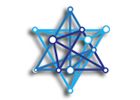# הדוקטורנטית תמר פורמן זכתה במלגה ע"ש יובל נאמןברכות לדוקטורנטית בחוג ללקויות למידה, בהנחייתה של ד"ר אורלי רובינשטיין שזכתה במלגה היוקרתית על שם יובל נאמן של משרד המדע, הטכולוגיה והחלל.
עבודת הדוקטורט שהוגשה למלגה עוסקת באופרציות לא סימבוליות מוערכות, אופרציות סימבוליות מוערכות ומדויקות ויכולת מתמטית.

תקציר העבודה:

Numerical representations are thought to rest on two representation systems: symbolic (e.g., Arabic numerals such as “6” or number words such as “SIX”) and non-symbolic approximate (ANS) representation (e.g., a group of dots). The fact that these representations are related is well documented. However, the literature is inconclusive regarding their relationship with mathematical proficiency. ANS is commonly examined by comparison tasks (two arrays of dots are presented and one is required to compare them), but recently some studies have focused on the performance of math operations (i.e., addition, subtraction and multiplication) within the ANS and approximately using symbolic representations.

In the current study I will try to answer and expand the question regarding the relation between the ANS and mathematical proficiency by focusing on three types of mathematical operations: (1) ANS operations (e.g., adding groups of dots and estimating their sum), (2) approximate symbolic operations (e.g., estimating the sum of 37+41), and (3) exact symbolic operations (e.g., 7+8=?). Their relation among themselves and with mathematical proficiency will be examined in five age groups.

This will be the first study to (1) examine these three types of operations (and one of the few that examine approximate symbolic ones) (2) examine their relation to mathematical proficiency, in (3) five different age groups. The outcomes of the current study may potentially enable the generation of new strategies in mathematics education, strategies that will utilize children’s ability to perform approximate mathematical operations in order to encourage the acquisition of mathematical knowledge.## קול קורא

משרות פתוחות (עבור סגל ומתעניינים אחרים) ומלגות (עבור סטודנטים) בפקולטה לחינוך